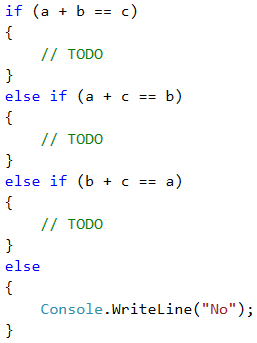Problem: Sums of 3 Numbers

The next sample exam problem is about checking multiple cases to find out if from given 3 numbers we can find two of them which sum up to the third of them.

Video: Sum of 3 Numbers

Watch the video lesson about solving the "Sum of 3 Numbers" problem: https://youtu.be/7NwKXOtbWXc.

Problem Description

There are 3 integers given. Write a program that checks if the sum of two of the numbers is equal to the third one. For example, if the numbers are 3, 5 and 2, the sum of two of the numbers is equal to the third one: 2 + 3 = 5.

Input

The console reads three integers, one per line. The numbers are in the range [1 … 1000].

Output

• Print a text line on the console containing the solution of the problem in the format "a + b = c", where a, b and c are among the three input numbers and a ≤ b.
• If the problem has no solution, print "No" on the console.

Sample Input and Output

Input Output Input Output
3
5
2
2 + 3 = 5 2
2
4
2 + 2 = 4
Input Output Input Output
1
1
5
No 2
6
3
No

We take the input from the console.Composing a Template for the Solution

We have to check if the sum of a pair of numbers is equal to the third number. We have three possible cases:

• a + b = c
• a + c = b
• b + c = a

We will write a template, which will later be complemented by the required code. If none of the above three conditions is met, we will make our program print "No".Writing Code in the Template

We now have to understand the order in which the two addends will be written in the output of the program. For this purpose, we will create a nested condition that checks which one of the two numbers is the larger one. In the first case, it will look like this:Similarly, we will supplement the other two cases. The full code of the program will look like this: